# Conduct and Interpret a Cluster Analysis

What is the Cluster Analysis?

Cluster analysis is an exploratory analysis that tries to identify structures within the data.  Cluster analysis is also called segmentation analysis or taxonomy analysis.  More specifically, it tries to identify homogenous groups of cases if the grouping is not previously known.  Because it is exploratory, it does not make any distinction between dependent and independent variables.  The different cluster analysis methods that SPSS offers can handle binary, nominal, ordinal, and scale (interval or ratio) data.

Cluster analysis is often used in conjunction with other analyses (such as discriminant analysis).  The researcher must be able to interpret the cluster analysis based on their understanding of the data to determine if the results produced by the analysis are actually meaningful.

Typical research questions the cluster analysis answers are as follows:

• Medicine – What are the diagnostic clusters?  To answer this question the researcher would devise a diagnostic questionnaire that includes possible symptoms (for example, in psychology, anxiety, depression etc.).  The cluster analysis can then identify groups of patients that have similar symptoms.
• Marketing – What are the customer segments?  To answer this question a market researcher may conduct a survey covering needs, attitudes, demographics, and behavior of customers.  The researcher then may use cluster analysis to identify homogenous groups of customers that have similar needs and attitudes.
• Education – What are student groups that need special attention?  Researchers may measure psychological, aptitude, and achievement characteristics.  A cluster analysis then may identify what homogeneous groups exist among students (for example, high achievers in all subjects, or students that excel in certain subjects but fail in others).
• Biology – What is the taxonomy of species?  Researchers can collect a data set of different plants and note different attributes of their phenotypes.  A cluster analysis can group those observations into a series of clusters and help build a taxonomy of groups and subgroups of similar plants.

Other techniques you might want to try in order to identify similar groups of observations are Q-analysis, multi-dimensional scaling (MDS), and latent class analysis.

The Cluster Analysis in SPSS

Our research question for this example cluster analysis is as follows:

What homogenous clusters of students emerge based on standardized test scores in mathematics, reading, and writing?

In SPSS Cluster Analyses can be found in Analyze/Classify….  SPSS offers three methods for the cluster analysis: K-Means Cluster, Hierarchical Cluster, and Two-Step Cluster.

K-means cluster is a method to quickly cluster large data sets.  The researcher define the number of clusters in advance.  This is useful to test different models with a different assumed number of clusters.

Hierarchical cluster is the most common method.  It generates a series of models with cluster solutions from 1 (all cases in one cluster) to n (each case is an individual cluster).  Hierarchical cluster also works with variables as opposed to cases; it can cluster variables together in a manner somewhat similar to factor analysis.  In addition, hierarchical cluster analysis can handle nominal, ordinal, and scale data; however it is not recommended to mix different levels of measurement.

Two-step cluster analysis identifies groupings by running pre-clustering first and then by running hierarchical methods.  Because it uses a quick cluster algorithm upfront, it can handle large data sets that would take a long time to compute with hierarchical cluster methods.  In this respect, it is a combination of the previous two approaches.  Two-step clustering can handle scale and ordinal data in the same model, and it automatically selects the number of clusters.The hierarchical cluster analysis follows three basic steps: 1) calculate the distances, 2) link the clusters, and 3) choose a solution by selecting the right number of clusters.

First, we have to select the variables upon which we base our clusters.  In the dialog window we add the math, reading, and writing tests to the list of variables.  Since we want to cluster cases we leave the rest of the tick marks on the default.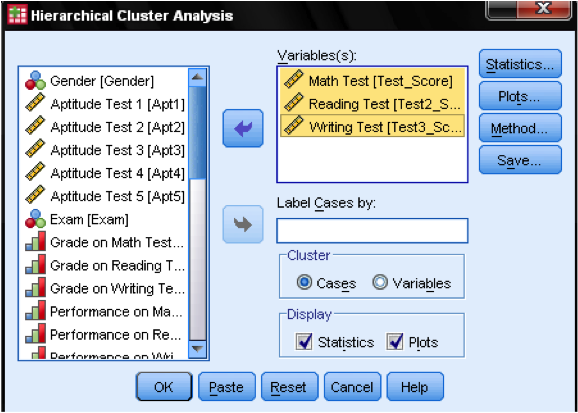In the dialog box Statistics… we can specify whether we want to output the proximity matrix (these are the distances calculated in the first step of the analysis) and the predicted cluster membership of the cases in our observations.  Again, we leave all settings on default.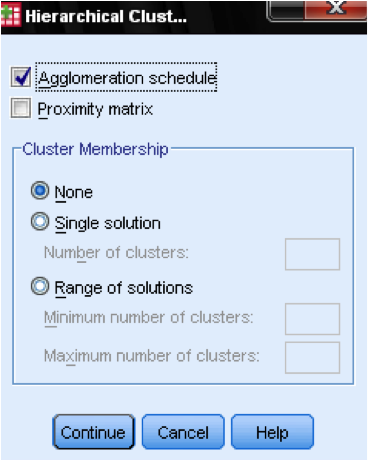In the dialog box Plots… we should add the Dendrogram.  The Dendrogram will graphically show how the clusters are merged and allows us to identify what the appropriate number of clusters is.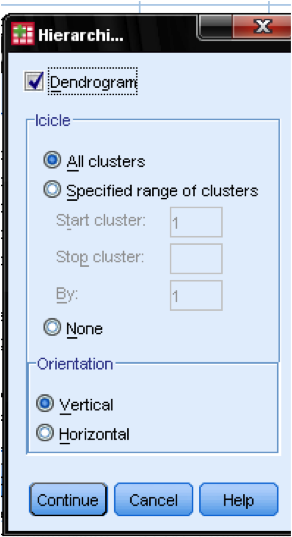The dialog box Method… allows us to specify the distance measure and the clustering method.  First, we need to define the correct distance measure.  SPSS offers three large blocks of distance measures for interval (scale), counts (ordinal), and binary (nominal) data.

For interval data, the most common is Square Euclidian Distance.  It is based on the Euclidian Distance between two observations, which is the square root of the sum of squared distances.  Since the Euclidian Distance is squared, it increases the importance of large distances, while weakening the importance of small distances.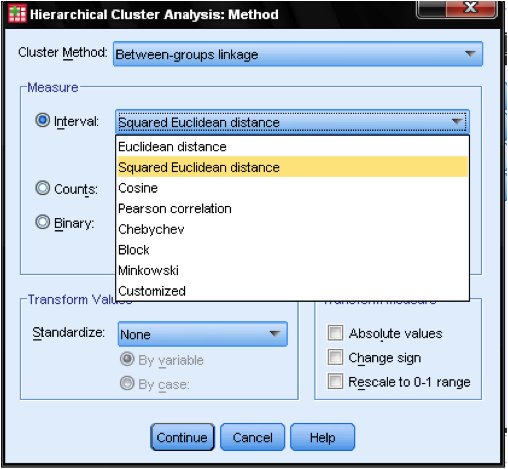If we have ordinal data (counts) we can select between Chi-Square or a standardized Chi-Square called Phi-Square.  For binary data, the Squared Euclidean Distance is commonly used.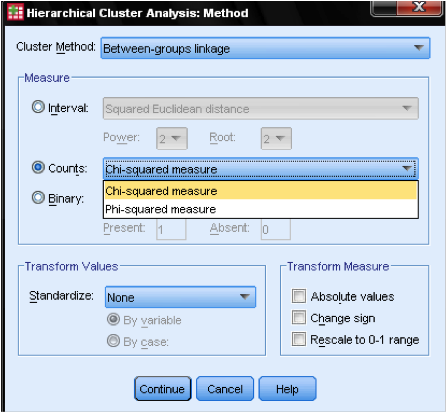In our example, we choose Interval and Square Euclidean Distance.Next, we have to choose the Cluster Method.  Typically, choices are between-groups linkage (distance between clusters is the average distance of all data points within these clusters), nearest neighbor (single linkage: distance between clusters is the smallest distance between two data points), furthest neighbor (complete linkage: distance is the largest distance between two data points), and Ward’s method (distance is the distance of all clusters to the grand average of the sample).  Single linkage works best with long chains of clusters, while complete linkage works best with dense blobs of clusters.  Between-groups linkage works with both cluster types.  It is recommended is to use single linkage first.  Although single linkage tends to create chains of clusters, it helps in identifying outliers.  After excluding these outliers, we can move onto Ward’s method.  Ward’s method uses the F value (like in ANOVA) to maximize the significance of differences between clusters.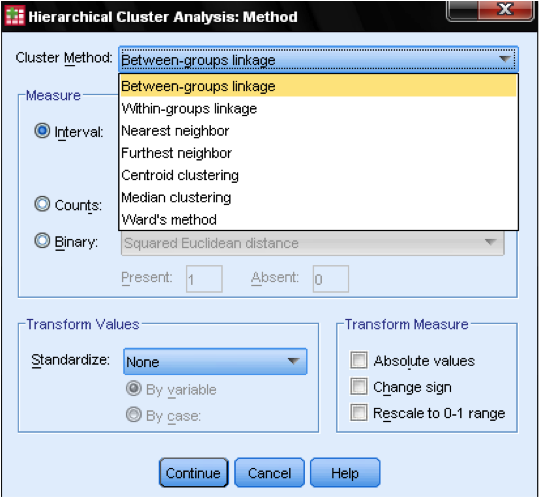A last consideration is standardization.  If the variables have different scales and means we might want to standardize either to Z scores or by centering the scale.  We can also transform the values to absolute values if we have a data set where this might be appropriate.